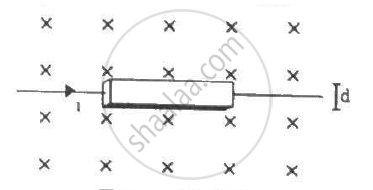Advertisement Remove all ads

# A Current I is Passed Through a Silver Strip of Width D and Area of Cross-section A. the Number of Free Electrons per Unit Volume is N. - Physics

Sum

A current i is passed through a silver strip of width d and area of cross-section A. The number of free electrons per unit volume is n. (a) Find the drift velocity v of the electrons. (b) If a magnetic field B exists in the region, as shown in the figure, what is the average magnetic force on the free electrons? (c) Due to the magnetic force, the free electrons get accumulated on one side of the conductor along its length. This produces a transverse electric field in the conductor, which opposes the magnetic force on the electrons. Find the magnitude of the electric field which will stop further accumulation of electrons. (d) What will be the potential difference developed across the width of the conductor due to the electron-accumulation? The appearance of a transverse emf, when a current-carrying wire is placed in a magnetic field, is called Hall effect.Advertisement Remove all ads

#### Solution

Given:-

Width of the silver strip = d

Area of cross-section = A

Electric current flowing through the strip = i

The number of free electrons per unit volume = n

(a) The relation between the drift velocity and current through any wire,

i = vnAe, where e = charge of an electron and v is the drift velocity.

v = i/(nAe)

(b)The magnetic field existing in the region is B.

The average magnetic force on a current-carrying conductor,

F = ilB

So, the force on a free electron  = (ilb)/(nAl) = (iB)/(nA)upwards (Using Fleming's left-hand rule)

(c) Let us take the electric field as E.

So, further accumulation of electrons will stop when the electric force is just balanced by the magnetic force.

eF= F =(iB)/(An)

⇒ E =(iB)/(eAn)

(d) The potential difference developed across the width of the conductor due to the electron-accumulation will be

V = Er

⇒ E × d = (iBd)/(eAn)

Concept: Force on a Moving Charge in Uniform Magnetic and Electric Fields
Is there an error in this question or solution?
Advertisement Remove all ads

#### APPEARS IN

HC Verma Class 11, Class 12 Concepts of Physics Vol. 2
Chapter 12 Magnetic Field
Q 29 | Page 232
Advertisement Remove all ads
Advertisement Remove all ads
Share
Notifications

View all notifications

Forgot password?